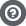Hello Guest

• 4 Replies
• 3852 Views####Raindeer

•• 5« on: June 20, 2013, 18:07:23 »
Hello!

I'm new to these forums and I have run into a problem. I can load the sprites from the atlas completely fine, but the problem occurs when I try to bind the BufferedImage texture, which is not supported. So, I load the atlas, get the subimages but I can't bind them as textures. Is there a way to convert a BufferedImage to a working texture without 500 packages of premade code? Well, if you have any good solutions for this problem it'll certainly be appreciated.

Thank you.
« Last Edit: June 21, 2013, 08:25:47 by Raindeer »####Fool Running

•• 828« Reply #1 on: June 20, 2013, 20:59:34 »
I think you misunderstand how to use a texture atlas in OpenGL. You create an OpenGL texture using the whole atlas. Then you just adjust the texture coordinates used to select the parts of the atlas making up the sprite.

If you need a simple, easy-to-use texture loader, I would suggest the one in Slick Utils: http://slick.ninjacave.com/slick-util/
Programmers will, one day, rule the world... and the world won't notice until its too late.Just testing the marquee option####Raindeer

•• 5« Reply #2 on: June 20, 2013, 22:09:40 »
I think you misunderstand how to use a texture atlas in OpenGL. You create an OpenGL texture using the whole atlas. Then you just adjust the texture coordinates used to select the parts of the atlas making up the sprite.

If you need a simple, easy-to-use texture loader, I would suggest the one in Slick Utils: http://slick.ninjacave.com/slick-util/

I actually did that as a placeholder, but things didn't work out quite well. The sprites interfere with each other, the edges' colors mix together and it looks really bad. And I am using slick utils. The sprites are 16x16 and are set to GL_NEAREST, pixelated textures, like in Minecraft. Dunno if the sprite resolution has anything to do with it.####Raindeer

•• 5« Reply #3 on: June 21, 2013, 08:10:46 »
This is the code for drawing a cuboid.

Code: [Select]
`import org.lwjgl.opengl.GL11;public class PolyVoxel extends Poly { float x1, y1, z1, x2, y2, z2, centerX, centerY, centerZ; float width, height, length; float yTop, yBottom; private float tx1, ty1, tx2, ty2; private int textureX, textureY; public PolyVoxel(float startX, float startY, float startZ, float endX, float endY, float endZ, int spriteX, int spriteY) { x1 = startX; y1 = startY; z1 = startZ; x2 = endX; y2 = endY; z2 = endZ; width = x2 - x1; height = y2 - y1; length = z2 - z1; centerX = x1 + (width/2); centerY = y1 + (height/2); centerZ = z1 + (length/2); textureX = spriteX; textureY = spriteY; tx1 = (float) spriteX / 32f; ty1 = (float) spriteY / 32f; tx2 = tx1 + (1f / 32f); ty2 = ty1 + (1f / 32f); System.out.println(String.format("%s, %s, %s, %s", tx1, ty1, tx2, ty2)); } public void render() { GL11.glBegin(GL11.GL_QUADS); { //top GL11.glTexCoord2f(tx1, ty1); GL11.glVertex3f(x1, y1, z1); GL11.glTexCoord2f(tx2, ty1); GL11.glVertex3f(x1+width, y1, z1); GL11.glTexCoord2f(tx2, ty2); GL11.glVertex3f(x1+width, y1, z1+length); GL11.glTexCoord2f(tx1, ty2); GL11.glVertex3f(x1, y1, z1+length); //bottom GL11.glTexCoord2f(tx1, ty1); GL11.glVertex3f(x1, y1+height, z1); GL11.glTexCoord2f(tx2, ty1); GL11.glVertex3f(x1+width, y1+height, z1); GL11.glTexCoord2f(tx2, ty2); GL11.glVertex3f(x1+width, y1+height, z1+length); GL11.glTexCoord2f(tx1, ty2); GL11.glVertex3f(x1, y1+height, z1+length); //front GL11.glTexCoord2f(tx1, ty1); GL11.glVertex3f(x1, y1, z1); GL11.glTexCoord2f(tx2, ty1); GL11.glVertex3f(x1+width, y1, z1); GL11.glTexCoord2f(tx2, ty2); GL11.glVertex3f(x1+width, y1+height, z1); GL11.glTexCoord2f(tx1, ty2); GL11.glVertex3f(x1, y1+height, z1); //back GL11.glTexCoord2f(tx1, ty1); GL11.glVertex3f(x1, y1, z1+length); GL11.glTexCoord2f(tx2, ty1); GL11.glVertex3f(x1+width, y1, z1+length); GL11.glTexCoord2f(tx2, ty2); GL11.glVertex3f(x1+width, y1+height, z1+length); GL11.glTexCoord2f(tx1, ty2); GL11.glVertex3f(x1, y1+height, z1+length); //left GL11.glTexCoord2f(tx1, ty1); GL11.glVertex3f(x1, y1, z1); GL11.glTexCoord2f(tx2, ty1); GL11.glVertex3f(x1, y1, z1+length); GL11.glTexCoord2f(tx2, ty2); GL11.glVertex3f(x1, y1+height, z1+length); GL11.glTexCoord2f(tx1, ty2); GL11.glVertex3f(x1, y1+height, z1); //right GL11.glTexCoord2f(tx1, ty1); GL11.glVertex3f(x1+width, y1, z1); GL11.glTexCoord2f(tx2, ty1); GL11.glVertex3f(x1+width, y1, z1+length); GL11.glTexCoord2f(tx2, ty2); GL11.glVertex3f(x1+width, y1+height, z1+length); GL11.glTexCoord2f(tx1, ty2); GL11.glVertex3f(x1+width, y1+height, z1); } GL11.glEnd(); } public int getTextureX() { return textureX; } public int getTextureY() { return textureY; } public void setTexture(int texX, int texY) { textureX = texX; textureY = texY; tx1 = (float) texX / 32f; ty1 = (float) texY / 32f; tx2 = tx1 + (1f / 32f); ty2 = ty1 + (1f / 32f); }}`
So the edges of the textures interfere, mixing the colors up. Here's the spritesheet:And makes it turn out like this:####Raindeer

•• 5